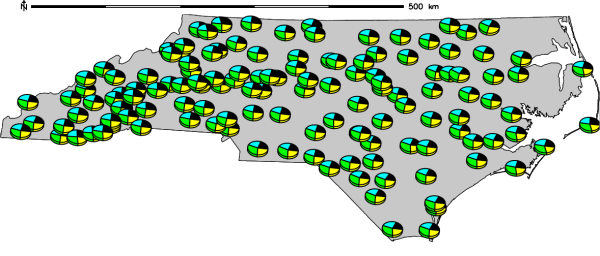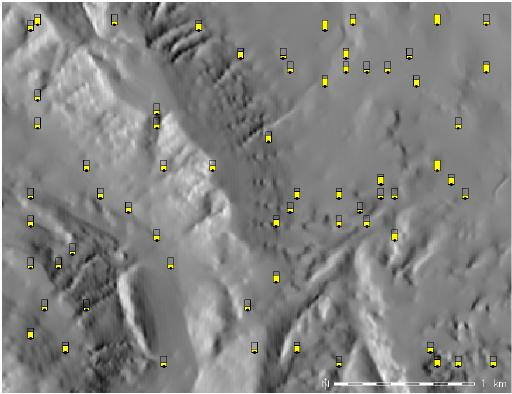Note: This document is for an older version of GRASS GIS that will be discontinued soon. You should upgrade, and read the current manual page.

## NAME

d.vect.chart - Displays charts of vector data in the active frame on the graphics monitor.

## KEYWORDS

display, cartography, chart maps

## SYNOPSIS

d.vect.chart
d.vect.chart --help
d.vect.chart [-cl3] map=name [type=string[,string,...]] [layer=string] [chart_type=string] columns=name[,name,...] [size_column=name] [size=integer] [scale=float] [outline_color=name] [colors=name[,name,...]] [max_ref=float[,float,...]] [--help] [--verbose] [--quiet] [--ui]

### Flags:

-c
Center the bar chart around a data point
-l
Create legend information and send to stdout
-3
Create 3D charts
--help
Print usage summary
--verbose
Verbose module output
--quiet
Quiet module output
--ui
Force launching GUI dialog

### Parameters:

map=name [required]
Name of vector map
Or data source for direct OGR access
type=string[,string,...]
Input feature type
Options: point, line, boundary, centroid, area
Default: point,line,boundary,centroid
layer=string
Layer number or name
Vector features can have category values in different layers. This number determines which layer to use. When used with direct OGR access this is the layer name.
Default: 1
chart_type=string
Chart type
Options: pie, bar
Default: pie
columns=name[,name,...] [required]
Attribute columns containing data
size_column=name
Column used for pie chart size
size=integer
Size of chart (diameter for pie, total width for bar)
Default: 40
scale=float
Scale for size (to get size in pixels)
Default: 1
outline_color=name
Outline color
Either a standard color name or R:G:B triplet
Default: black
colors=name[,name,...]
Colors used to fill charts
Either a standard color name or R:G:B triplet
Default: black
max_ref=float[,float,...]
Maximum value used for bar plot reference

## DESCRIPTION

d.vect.chart displays charts for GRASS vector data in the active frame on the graphics monitor.

## NOTES

The charts are positioned as follows:
• vector points: on point position,
• vector lines: on line centers,
• vector areas: on area centroids.
Bar charts are placed with their lower edge starting from the y-coordinate of the feature being symbolized, and centered with respect to the x-coordinate. The -c flag can be used to center the bar chart in both x and y directions.

The 'sizecol' parameter is proportionate to the radius.

The optional max_ref parameter accepts a list of values that represent the maximum value for each column listed in the values for the parameter columns. These values are used to create a framed bar plot if chart_type is bar (See Example 2).

## EXAMPLES

### Example 1

Pie-charts of monthly winter precipitation (North Carolina sample dataset):
```g.region vector=nc_state -p
d.vect nc_state
d.vect.chart precip_30ynormals chart_type=pie columns=nov,dec,jan,feb -l

# show pie chart as 3D
d.erase
d.vect nc_state
d.vect.chart precip_30ynormals chart_type=pie columns=nov,dec,jan,feb -l -3
```2D pie-chart of monthly winter precipitation in North Carolina3D pie-chart of monthly winter precipitation in North Carolina

### Example 2

Bar-chart of different census map values:
```d.vect.chart map=vectmap columns=cens51,cens61,cens71,cens81 chart_type=bar
```

### Example 3

Creation of framed bar charts of an erodibiliy index from the Spearfish sample dataset:
```g.region raster=erode.index -p
r.to.vect -s input=erode.index output=erode_index type=area
v.extract input=erode_index output=erode_index_ctrds type=centroid
d.rast aspect
d.vect.chart map=erode_index_ctrds chart_type=bar columns=cat \
size=10 max_ref=12 scale=0.05 colors=yellow
d.vect erode_index_ctrds icon=basic/circle fcol=black col=black size=5
```Bar charts of an erodibiliy index (spatial subset)

d.erase, d.vect, d.vect.thematic, d.what.vect, d.rast